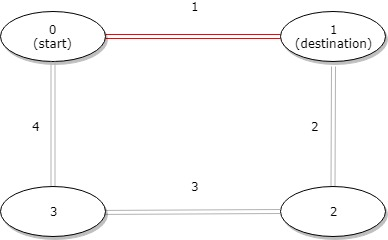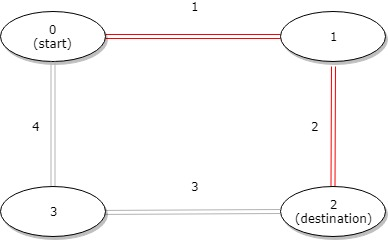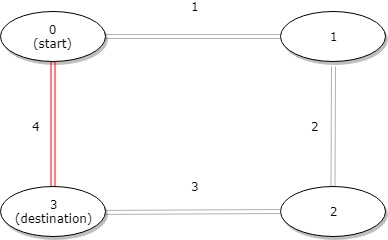LeetCode: 1184. 公交站间的距离¶

1、题目描述¶• 1 <= n <= 10^4
• distance.length == n
• 0 <= start, destination < n
• 0 <= distance[i] <= 10^4

2、解题思路¶

• 只需要判断两站之间和两站之外那个距离更短即可
class Solution:
def distanceBetweenBusStops(self, distance: List[int], start: int, destination: int) -> int:
start, destination = (start, destination) if start < destination else (destination, start)
total = sum(distance)
between = sum(distance[start:destination])
return min(between, total - between)